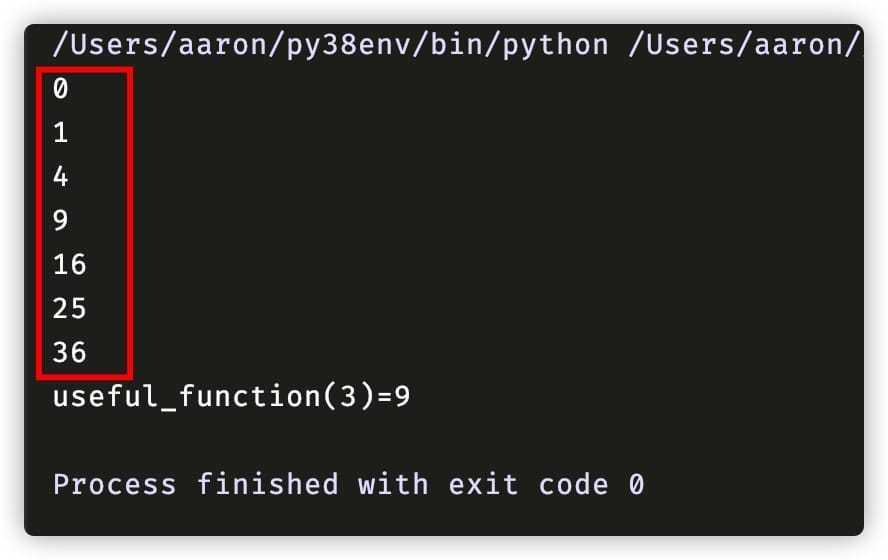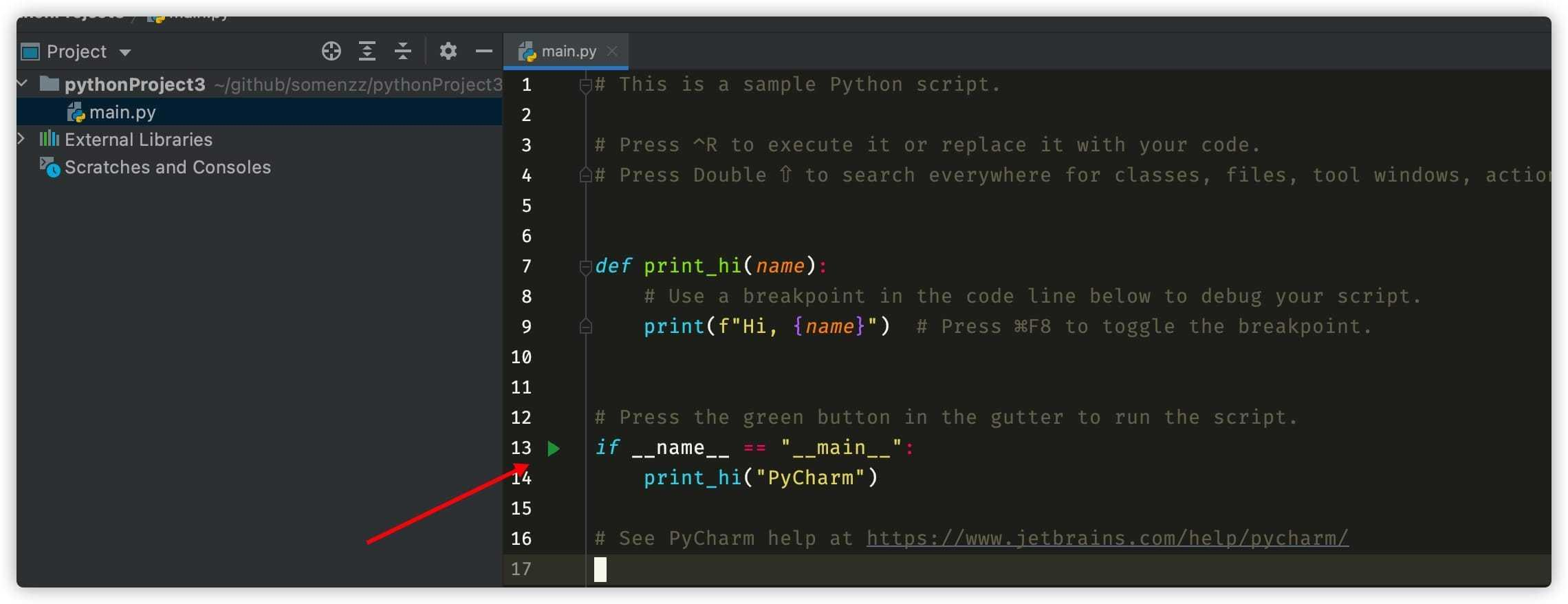# 写Python脚本，一定要加上这个

```python def main(): # do something print("do something.")

if name == "main": main() ```

## 第一，它让 Python 文件的作用更加明确

```python print("some_script.py") print(__name__)```

```python ❯ vim some_script.py ❯ python Python 3.8.5 (v3.8.5:580fbb018f, Jul 20 2020, 12:11:27) [Clang 6.0 (clang-600.0.57)] on darwin Type "help", "copyright", "credits" or "license" for more information.

import some_script some_script.py some_script

````` 可以看到，```name` 的值就是 Python 脚本的文件名 some_script。

```python def useful_function(x): return x * x

class UsefulClass: def init(self, x): self.x = x

# 你自己测试了一吧，没毛病

for i in range(7): print(useful_function(i)) ```

def main(): print(f'{useful_function(3)=}')

if name == 'main': main() ```## 第二，它让 Python 文件更加易读，对 IDE 友好`if __name__ == "__main__":` 的那一行的最左边也有一个绿色的运行按钮，点击一下，程序就从这一行开始运行了。

## 第三、多进程场景下，必须用 if main

```python import multiprocessing as mp

def useful_function(x): return x * x

print("processing in parallel") with mp.Pool() as p: results = p.map(useful_function, [1, 2, 3, 4]) print(results)

```

```python import multiprocessing as mp

def useful_function(x): return x * x

if name == 'main': print("processing in parallel") with mp.Pool() as p: results = p.map(useful_function, [1, 2, 3, 4]) print(results) ```

## 最后的话

`if __name__ == "__main__":` 虽然不是强制的，但是基于上述三点原因，我强列推荐你这么做，它是 Python 社区的约定，对应Python 之禅：明确优于隐晦。正如 `_` 作为变量名的意思就是告诉读代码的人：这个变量不重要，后面也不会用到它。当你看到 Python 脚本有 `if __name__ == "__main__":` 时，就会意识到，这是一个可执行的脚本，当被其他程序导入时，这部分代码不会被执行，而多进程的程序中，这是必须的。

「其他文章」